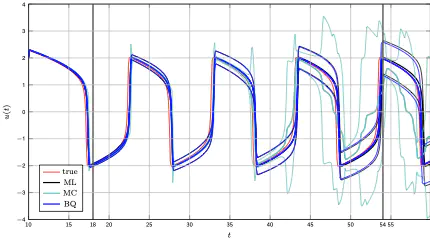# Active Uncertainty Calibration in Bayesian ODE SolversActive uncertainty calibration by Bayesian quadrature

### Abstract

There is resurging interest, in statistics and machine learning, in solvers for ordinary differential equations (ODEs) that return probability measures instead of point estimates. Recently, Conrad et al. introduced a sampling-based class of methods that are ‘well-calibrated’ in a specific sense. But the computational cost of these methods is significantly above that of classic methods. On the other hand, Schober et al. pointed out a precise connection between classic Runge–Kutta ODE solvers and Gaussian filters, which gives only a rough probabilistic calibration, but at negligible cost overhead. By formulating the solution of ODEs as approximate inference in linear Gaussian SDEs, we investigate a range of probabilistic ODE solvers, that bridge the trade-off between computational cost and probabilistic calibration, and identify the inaccurate gradient measurement as a crucial source of uncertainty. We propose the novel filtering-based method Bayesian Quadrature filtering (BQF) which uses Bayesian quadrature to actively learn the imprecision in the gradient measurement by collecting multiple gradient evaluations.

Type
Publication
Proceedings of the Thirty-Second Conference on Uncertainty in Artificial Intelligence##### Hans Kersting
###### Research Scientist

My research interests lie in machine learning, optimization, and data science.